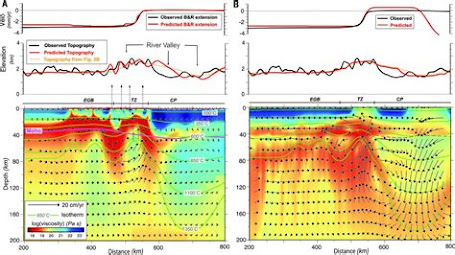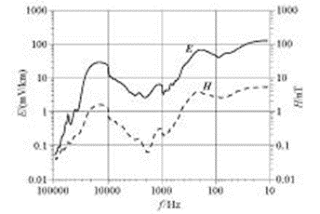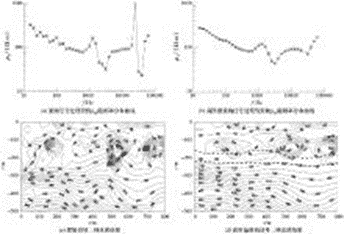High frequency magnetotelluric noise suppression

### Summary

The high-frequency magnetotelluric (HMT) signal is easily disturbed by human noise in the frequency range of 20 kHz to 100 kHz, the electromagnetic signal strength is weak, and the data error is large. This paper proposes to use high-order spectral analysis to reconstruct the high-frequency magnetotelluric non-minimum phase signal, thereby effectively suppressing random Gaussian noise and improving the signal-to-noise ratio. Through the processing of numerical simulation signal and measured data, it is proved that the method can effectively suppress the noise, and it is better than the traditional method in extracting the actual geological information.

Key words : high-frequency magnetotelluric method ; high-order spectrum ; signal processing ; noise suppression

## Abstract

In the frequency range of 20kHz ~ 100kHz, the HMT signal has the characteristics of weak strength, low correlation and poor repeatability, which is caused by the interference of human noise in the near surface. In this paper, the authors used higher-order spectrum method to analyze and reconstruct HMT signal, which can effectively suppress Gaussian random noise and raise signal noise ratio. Numerical analog signal and measured data processing prove that the method is feasible. According to the application effect, the method is trade better the colored noise suppression and practical geological information extraction.

Keyword : high frequency magnetotelluric method ; higher-order spectrum ; signal processing ; noise suppression

## 0 Preface

The high-frequency magnetotelluric method (HMT) was proposed according to the engineering geological exploration needs of deep-buried tunnels. The engineering survey is most concerned with the stratum geological conditions within 1000 m near the surface[  ] . It is 10 Hz~100 kHz, which is different from the traditional magnetotelluric (MT) observation frequency band of 0.001~340 Hz and the audio frequency magnetotelluric method (AMT) observation frequency band of 0.1 Hz~ n kHz [ ] . The electromagnetic method is distinguished, which is defined as the high-frequency magnetotelluric method. At present, the high-frequency magnetotelluric method can be realized by using the electromagnetic observation system EH-4 conductivity tensor measuring instrument jointly developed and produced by GEOMETRICS and EMI. In recent years, the author has selected nearly a hundred representative measuring points in several provinces and cities in southeastern China, measured the electromagnetic fields in the 10 Hz-100 kHz frequency band, and calculated the average amplitude strength of natural electromagnetic fields in southeastern China (Fig. 1 ). The results show that In the frequency range of 20 kHz to 100 kHz, the signal strength of the electric field and magnetic field weakens as a whole, and the correlation of the electromagnetic field signal decreases and the repeatability is poor. This is because high-frequency signals are easily interfered by human noise, such as high-voltage wires, electric railways, and telecommunications grounding equipment. The complex and diverse noise effects often make the repeatability of observations worse, and the estimation of impedance is scattered, which cannot objectively reflect the underground electrical properties. distribution, it may even lead to erroneous results.

Wang Shuming et al.  believe that the magnetotelluric signal is non-Gaussian, non-minimum phase and non-linear, that is, a "three non-" signal, and the classical spectrum estimation method loses the phase information, and is sensitive to high-frequency human noise suppression is not ideal. The author tried to reconstruct the original time series by using the high-order spectral estimation method to suppress the colored Gaussian noise, and then calculate the resistivity and phase. From the perspective of application effect, this method is better than the traditional method in suppressing the colored noise and extracting the actual geological information.Figure 1 The average amplitude of high-frequency electromagnetic field strength in southeastern China

### 1 Analysis of high-order spectrum of HMT signal

Suppose the signal x ( k ) is a k -th order stationary random process with zero mean , then the k -th order moment kx ( τ 1 , ..., τ k- 1 ) of the process is defined as

The k- order cumulant of the process ( τ 1 , …, τ k- 1 ) is defined as

where: k ≥ 3, g ( n ) is a Gaussian process with the same power spectrum as x ( n ).

Thus, the third-order cumulant of x ( k ) signal is

where E { } represents mathematical expectation. After Fourier transform, formula (1) becomes

(2)

Equation (2) is the third-order spectrum of x ( k ) , also called bispectrum, where ω the frequency. The third-order spectrum has symmetry, namely:

From formula (2), we can know that the phase spectrum of the bispectrum is

#### The amplitude spectrum is

where ϕ x ( ω ) and |X ( ω ) | are the phase spectrum and amplitude spectrum of x ( k ) , respectively .

2 High-order spectrum estimation to reconstruct the power spectrum

## 2.1 Amplitude estimation

By taking the logarithm on both sides of formula (5), we can get

Substituting equation (6) as a variable, that is, ω +ω =i , ω =j , ω =ij , the discrete expression can be obtained

When taking i= 0, j= 0, we can get

### 2.2 Phase Estimation

There are many methods for bispectrum phase estimation [ ] , and the BMU algorithm is used in this paper. Brillinger first proposed a method to recursively calculate ϕ ( ω ) from ϕ ( ω 1 , ω 2 ) , the starting point relation is

In order to facilitate the operation and improve the calculation accuracy, Matsuoka and Ulrych derived the discrete form of equation (10), in the region

ω 1 + ω ω (0≤ω ≤ω ; 0≤ω ≤ω ) , further in the ω = [0, ω ] summation,

let ω =ω -ω 1 get

For the convenience of calculation, let Δ ω = 1, ω =i , ω =j , ω =n , we can get

(11)

in,

therefore,

Change the above formula into recursive form, define

then there is

where n=N corresponds to ω = π. The initial value of the formula is

So far, we have extracted the amplitude and phase from the third-order spectrum of the magnetotelluric signal, obtained the signal spectrum, reconstructed the signal through inverse Fourier transform, and then estimated the power spectrum to obtain the impedance tensor components, apparent Resistivity and phase information.

## 3 Theoretical and measured data processing and analysis

### 3.1 Numerical simulation analysis

The effect of high-order spectral technique on suppressing random noise of HMT signal is tested by numerical simulation synthetic data. Digital simulation of high-frequency magnetotelluric high-frequency signals: the number of sampling points is 4096, and the sampling frequency is 12 kHz, which contains sinusoidal signals with frequencies of 30, 50, 100, 200, 300, 1000, and 2000 Hz, using the matlab program rpiid function Gaussian noise, nonlinear drift and DC components are added.

Figure 2a is the synthetic signal of numerical simulation, and Figure 2b ~d are the amplitude spectrum and phase spectrum of the Fourier transform of the original signal and the reconstructed signal, respectively. It can be seen from Fig. 2b that although the original signal can distinguish 7 fundamental frequencies through Fourier transform, its anti-interference performance is very poor, and the frequency spectrum above 100 Hz is seriously disturbed by noise, among which 1 000 and 2 000 Hz are basically submerged in the in the noise. It can be seen from Fig. 2d that the phase spectrum noise of the original signal is severely interfered, and it is basically impossible to effectively distinguish each fundamental frequency. Since the high-order statistic of Gaussian noise is 0, the signal reconstructed through high-order spectrum is an effective means to suppress Gaussian noise. Fig. 2c and e are the corresponding Fourier transform amplitude spectra of signal reconstructed through high-order spectrum, The phase spectrum can accurately and clearly distinguish the designed 7 fundamental frequencies.

Through numerical signal simulation, it can be proved that the high-order spectral reconstruction signal can suppress random Gaussian noise and improve the signal-to-noise ratio of data.

### 3.2 Processing results of measured data

The actual data collected by magnetotelluric sounding often have discrete frequency points and large error bars. The current data processing software cannot effectively suppress random interference noise and the unsteady characteristics of magnetotelluric signals are one of the reasons for this phenomenon. It can be seen from the simulation that the high-order spectral reconstruction signal technology has obvious effects in suppressing Gaussian noise and improving the signal-to-noise ratio [ ] . Fig. 3 shows the basic idea of ​​magnetotelluric data processing using high-order spectral signal reconstruction technology.

Figure 4 is a comparison of the results obtained by reconstructing the time series of the measured data through the high-order spectrum and the traditional processing results. Figure 4a shows the measured magnetotelluric signal of a certain tunnel. The resistivity curve presents saw-tooth fluctuations in the 10-100 Hz and 2 MHz-10 MHz bands, especially in the 2 MHz-10 MHz band, where high and low resistivity appear alternately. The data at this point has large dispersion and large error bars, and the data quality is unreliable. Figure 4b shows the high-order spectrum reconstruction of the magnetotelluric signal, the overall trend of the curve is basically consistent with that of Figure 3a , but its frequency point data is evenly and smoothly distributed, the data does not have obvious jumps, and the error is also within the allowable range, especially in the The high impedance caused by random Gaussian noise is obviously eliminated in the 2M~10MHZ frequency band, and the data quality is obviously better than the original signal. Figure 4c shows the result of traditional data processing. The resistivity contours are rather messy, and there are "bull's-eye" high-resistance closed circles in the shallow surface and deep parts, and it is basically impossible to effectively extract geological features. Figure 4d shows the magnetotelluric signal reconstructed by the high-order spectrum, the contour distribution characteristics are consistent with those in Figure 4c , but the contour distribution is smooth and smooth, which largely removes the local “bull’s-eye” on the shallow surface and deep "like" high-resistance closed-loop phenomenon. In Fig. 4d , the distribution of resistivity sections is relatively stable in the horizontal direction, which is consistent with the macroscopic geological background of sedimentary rocks. In the vertical direction, the overall trend is high-low-resistivity-high resistance, which can effectively distinguish the coal measures in the Permian sandstone. strata ( shown by the dotted line in Fig. 4d), it is speculated that the depth of the coal seam roof is about 170 m underground, which is consistent with the depth revealed by later drilling.Figure 4 Comparison of data processing results between measured original signal and reconstructed signal

## 4 Conclusions and recommendations

For high-frequency magnetotelluric signals in the frequency range of 20 kHz to 100 kHz, the strength of the electric field and magnetic field signals is generally weakened, and the correlation of electromagnetic field signals is reduced, susceptible to interference, and poor in repeatability. Through the processing and analysis of numerical synthetic data and measured data, the phenomenon of discrete frequency points and large error bars that often appear in apparent resistivity curves can be effectively suppressed, random Gaussian noise can be effectively suppressed, and the signal-to-noise ratio can be improved.

There are still some problems in the reconstruction of magnetotelluric signals using high-order spectrum technology that need further research. For example, the realization method of high-order spectrum and the initial value selection of phase estimation will affect the final data processing results to varying degrees.